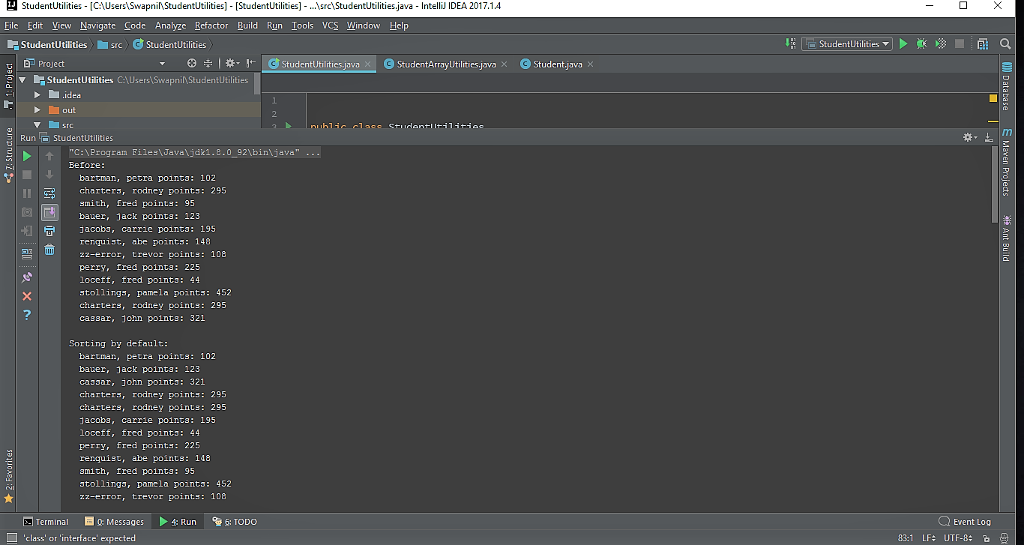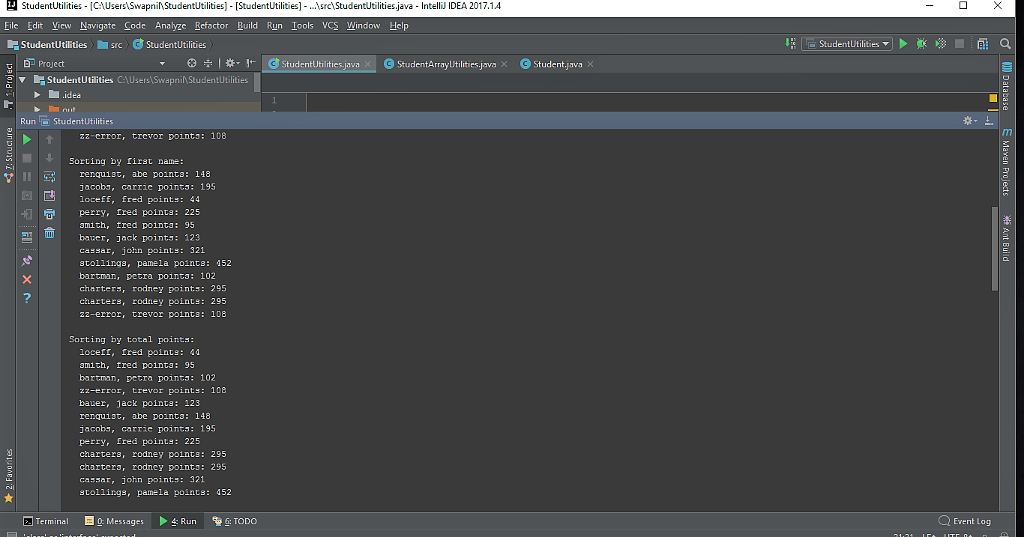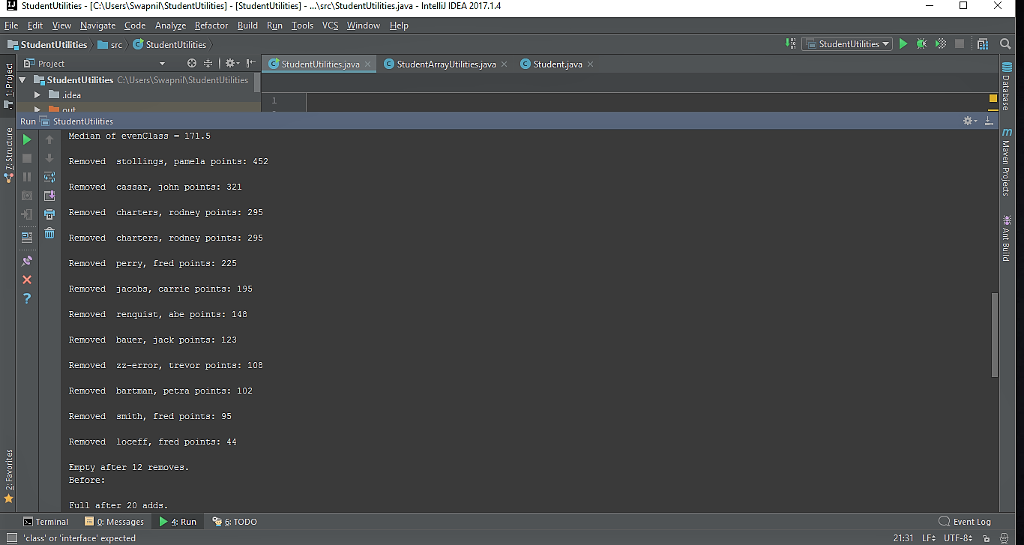# Question & Answer: Assignment 10 – A StudentArrayUtilities Class with an Internal Array…..

Assignment 10 – A StudentArrayUtilities Class with an Internal Array

Understand the Problem

Don't use plagiarized sources. Get Your Custom Essay on
Question & Answer: Assignment 10 – A StudentArrayUtilities Class with an Internal Array…..
GET AN ESSAY WRITTEN FOR YOU FROM AS LOW AS \$13/PAGE

Our previous StudentArrayUtilities (SAU) class consisted of a set of static class methods that acted on arrays of students. We take this a step further. Rather than having our client define and manage the array, let’s give SAU the ability to do that. There will be two main changes required:

Adding and Removing Students – We will provide methods addStudent() and removeStudent() as instance methods in our SAU class. Our clients can use these to add or remove individual students to an SAU object. The client may not see where or how the students are stored, internally. The extent to which we allow the client to gain access to the internal data is up to us, and in this assignment, we will give the client very limited access: our implementation will behave a bit like a stack data structure, so that adding is like “pushing” and removing is like (but not exactly the same as) “popping”.

Earlier Methods Become Instance Methods – Once we have the data internal to our SAU object, there is no need to pass arrays; the data is already present as this data. So older methods, like sort() and toString(), are converted to instance methods, and their signatures are changed to reflect this fact. When a sort is requested, the client only has to call someUtilObj.sort(), and it is understood that the Students to be sorted are the ones in the this object’s internal array created by the many previous calls to addStudent()and, perhaps, trimmed by some calls to removeStudent().

StudentArrayUtilities Spec

Summary

The class will store its private data as an array of Student references.   The natural — and in a senseuniversal — solution to this arrangement is to have two private data members: one for the array, and a second for the int which holds us the number of actual students being stored in the array at any point in time:

```   Student[] theArray;
int numStudents;
```

theArray will be a fixed-size array, that size being the maximum number of Studentswe expect to ever manage. It will hold many more elements than we need, typically.  numStudents would initially be 0(during an object instantiation of this class), would grow as students are added via addStudent() and shrink as Students are removed via removeStudent(). A common use for numStudents, besides telling us how many actual Students are in the object, is to tell any method that cares where the last (i.e., highest in the array) Student is stored. So, if numStudents is 12, it means there are 12 Students in the array, even if the theArray happens to have, say, 1000 = MAX_STUDENTS positions of available capacity.   The active Students are stored in locations 0 – 11theArray is the location of the Student in the highest occupied position, while theArray is where the next Student would be added if and when a subsequent call was made to addStudent().

The client may not need to know all this, but that’s what’s going on, internally.

An SAU object is instantiated using a default constructor and, once created, will use the addStudent()mutator to build a Student roster for the object. If the client wants to reduce the population of that object it would call removeStudent(). Here is a typical instantiation followed by the addition of a couple Students:

```   StudentArrayUtilities myStuds = new StudentArrayUtilities();

myStuds.addStudent( new Student("bartman", "petra", 102) );
```

As you see, there is no need for the client to create any arrays. However, if the client happens to have an array of Students, it can use that array along with a loop to add many students efficiently, as in:

```   for (k = 0; k < myClass.length; k++)
```

Once there are some Students in the SAU object, we can display them with the help of the instance method toString():

```    System.out.println( myStuds.toString("Here are the students currently being stored: "));
```

This is a method similar to the toString() of our older SAU class, but now we see no array need be passed, since this is an instance method which carries the full data of the object wherever it goes (via the this data). Likewise, the sort is handled without a parameter:

```    myStuds.arraySort();
```

That method call would result in our internal array being re-ordered. As before, and without any modifications needed, that sort would be based on the underlying Student class’s sort key.

Calling toString() after an arraySort() is invoked would naturally show the new order of the internal array.

Finally, the removeStudent() method would remove (from the internal array inside its SAU object) one of the Students. Which Student? That’s up to this spec and we could have many different answers. The answer for us (and for you) is that it will remove and return the student in the highest occupied array location of the internal array. So a call to:

```    student = myStuds.removeStudent();
```

Would remove the Student in location which is determined by the current value of the private member (but not necessarily that exact location — this is something you will determine based on all the information just given. It copies that removed object to the Student variable on the LHS for use by the client.

Static (Public) Members

A final int MAX_STUDENTS which you can set to 20 for testing, but would be larger in general. This is used to instantiate the internal array, whose capacity (physical array size) never changes from this one value.

Instance (Private) Members

Student[] theArray – our internal array whose size is always MAX_STUDENTS, but whose actual data is stored in elements 0 through numStudents – 1.

int numStudents – the current number of actual students stored in the array. This can never be >MAX_STUDENTS, and you have to make sure that it isn’t.

Instance (Public) Methods

boolean addStudent( Student stud ) – This method will place the passed-parameter into the next available location (highest) of our internal array. It has to test stud for null and also make sure not to overrun the internal array by breaching its capacity, MAX_STUDENTS. Returns false if error.

Student removeStudent() – Removes and returns the Student in the highest occupied position in the array. Note, depending on whether or not the internal array has been modified by a sort, this may — but does not have to be — the most recently added Student. If there are no students in the array, it returns null.

The following methods are still here, but are now changed to instance methods. The information that was supplied by their array parameters is now provided as part of the this object in the form of numStudents(for the array size) and theArray (for the hitherto passed array). You would now use those members in their definitions.

String toString( String title ) – This returns, usually for display by client, our the entire array in a singleString, just like the static version did, but without the need for an array parameter.

void arraySort() – Just like the old static sort, but no need for an array parameter.   It works on the internal array.

double getMedianDestructive() – as above.

Helper (Private) Methods

boolean floatLargestToTop( int top ) – Same as our old version, but now instance. Use this data in place of parameters lost from prior version.

Sample Client

Here is some client code to use while debugging. You should be able to determine the correct run that results. You should provide some code that is more complete than this in your testing.   For this test (and your own testing) set MAX_STUDENTSto 20.

```   public static void main (String[] args)
{
int k;
Student student;

Student[] myClass = { new Student("smith","fred", 95),
new Student("bauer","jack",123),
new Student("jacobs","carrie", 195),
new Student("renquist","abe",148),
new Student("3ackson","trevor", 108),
new Student("perry","fred",225),
new Student("loceff","fred", 44),
new Student("stollings","pamela",452),
new Student("charters","rodney", 295),
new Student("cassar","john",321),
};

// instantiate a StudArrUtilObject
StudentArrayUtilities myStuds = new StudentArrayUtilities();

// we can add stdunts manually and individually
myStuds.addStudent( new Student("bartman", "petra", 102) );

// if we happen to have an array available, we can add students in loop.
for (k = 0; k < myClass.length; k++)

System.out.println( myStuds.toString("Before: "));

myStuds.arraySort();
System.out.println( myStuds.toString("Sorting by default: "));

Student.setSortKey(Student.SORT_BY_FIRST);
myStuds.arraySort();
System.out.println( myStuds.toString("Sorting by first name: "));

Student.setSortKey(Student.SORT_BY_POINTS);
myStuds.arraySort();
System.out.println( myStuds.toString("Sorting by total points: "));

// test median
System.out.println("Median of evenClass = "
+  myStuds.getMedianDestructive() + "n");

// various tests of removing and adding too many students
for (k = 0; k < 100; k++)
{
if ( (student = myStuds.removeStudent()) != null)
System.out.println("Removed " + student);
else
{
System.out.println("Empty after " + k + " removes.");
break;
}
}

for (k = 0; k < 100; k++)
{
{
System.out.println("Full after " + k + " adds.");
break;
}
}
}
```

SAU and Student classes beforehand:

import javax.swing.*;
import java.util.*;

class Student
{
//sort keys
public static final int SORT_BY_FIRST = 88;
public static final int SORT_BY_LAST = 98;
public static final int SORT_BY_POINTS = 108;
private static int sortKey = SORT_BY_LAST; //setting to default

//members
private String lastName;
private String firstName;
private int totalPoints;

//constants
public static final String DEFAULT_NAME = ” [zz-error] “;
public static final int DEFAULT_POINTS = 0;
public static final int MAX_POINTS = 1000;

// constructor requires parameters – no default supplied
public Student(String last, String first, int points)
{
if ( !setLastName(last) )
lastName = DEFAULT_NAME;
if (!setFirstName(first))
firstName = DEFAULT_NAME;
if ( !setPoints(points) )
totalPoints = DEFAULT_POINTS;
}

//accessors
public String getLastName() { return lastName; }
public String getFirstName() { return firstName; }

//mutators
public boolean setLastName(String last)
{
if ( !validString(last) )
return false;
lastName = last;
return true;
}

public boolean setFirstName(String first)
{
if (!validString(first))
return false;
firstName = first;
return true;
}

public boolean setPoints(int pts)
{
if ( !validPoints(pts) )
return false;
totalPoints = pts;
return true;
}

// now looks at the sortKey and sorts students based on key
public static int compareTwoStudents(Student firstStud, Student secondStud)
{
switch(sortKey)
{
case SORT_BY_FIRST:
return firstStud.firstName.compareTo(secondStud.firstName);
case SORT_BY_LAST:
return firstStud.lastName.compareTo(secondStud.lastName);
case SORT_BY_POINTS:
return firstStud.totalPoints – secondStud.totalPoints;
default:
return 0;
}
}

//turns student into a readable string
public String toString()
{
String returnStr = lastName + “, ” + firstName + “. Points: ” + totalPoints;
return returnStr;
}

//mutator helper methods
private boolean validString( String testStr )
{
if (testStr != null && Character.isLetter(testStr.charAt(0)))
return true;
return false;
}

private boolean validPoints( int testPoints )
{
if (testPoints >= 0 && testPoints <= MAX_POINTS)
return true;
return false;
}

//sort key mutator
public static boolean setSortKey(int key)
{
switch(key)
{
case SORT_BY_FIRST:
case SORT_BY_LAST:
case SORT_BY_POINTS:
sortKey = key;
return true;
default:
return false;
}
}

//sort key accessor
public static int getSortKey() { return sortKey; }
}

class StudentArrayUtilities
{
//changed from printArray to toString, so it returns to client
public static String toString(String title, Student data[], int arraySize)
{
String output = title + ” n”;

for (int k = 0; k < arraySize; k++)
output += ” ” + data[k].toString() + “n”;

return output;
}

// returns true if a modification was made to the array
private static boolean floatLargestToTop(Student[] data, int top)
{
boolean changed = false;
Student temp;

// compare with client call to see where the loop stops
for (int k = 0; k < top; k++)
if ( Student.compareTwoStudents(data[k], data[k + 1]) > 0 )
{
temp = data[k];
data[k] = data[k + 1];
data[k + 1] = temp;
changed = true;
}
return changed;
}

//returns median of total scores of all students in the array
public static double getMedianDestructive(Student arr[], int arrSize)
{
int saveSortKey = Student.getSortKey(); //saving sortkey
double errorCatch = 400.0, returnVal; //in case it is not one of those cases, will return an error #
if (arrSize == 0) //checking empty array
return 0.0;
else if (arrSize == 1) //checking one person array
return arr.getTotalPoints();
else if (arrSize % 2 == 0) //checking even array
{
Student.setSortKey(Student.SORT_BY_POINTS);
returnVal = (arr[arrSize/2].getTotalPoints() + arr[arrSize/2 – 1].getTotalPoints())/2.0;
Student.setSortKey(saveSortKey);
return returnVal;
}
else if (arrSize % 2 == 1) //checking odd array
{
Student.setSortKey(Student.SORT_BY_POINTS);
returnVal = (arr[arrSize/2].getTotalPoints());
Student.setSortKey(saveSortKey);
return returnVal;
}
else
return errorCatch;
}

// public callable arraySort() – assumes Student class has a compareTo()
public static void arraySort(Student[] array)
{
for (int k = 0; k < array.length; k++)
// compare with method def to see where inner loop stops
if ( !floatLargestToTop(array, array.length – 1 – k) )
return;
}

public static int arraySearch(Student[] array,
String keyFirst, String keyLast)
{
for (int k = 0; k < array.length; k++)
if ( array[k].getLastName().equals(keyLast) )
return k; // found match, return index

return -1; // fell through – no match
}

public static int binarySearch(Student[] array, String keyLast, int firstIndex, int lastIndex)
{
int middleIndex, result;

if (firstIndex > lastIndex)
return -1;

middleIndex = (firstIndex + lastIndex) / 2;
result = keyLast.compareToIgnoreCase(array[middleIndex].getLastName());

if (result==0)
return middleIndex; //found him!
else if (result < 0)
return binarySearch( array, keyLast, firstIndex, middleIndex – 1);
else
return binarySearch( array, keyLast, middleIndex + 1, lastIndex);
}
}

StudentUtilities.java
—————————–

public class StudentUtilities
{
public static void main (String[] args)
{
int k;
Student student;

Student[] myClass = { new Student(“smith”,”fred”, 95),
new Student(“bauer”,”jack”,123),
new Student(“jacobs”,”carrie”, 195),
new Student(“renquist”,”abe”,148),
new Student(“3ackson”,”trevor”, 108),
new Student(“perry”,”fred”,225),
new Student(“loceff”,”fred”, 44),
new Student(“stollings”,”pamela”,452),
new Student(“charters”,”rodney”, 295),
new Student(“cassar”,”john”,321),
};

// instantiate a StudArrUtilObject
StudentArrayUtilities myStuds = new StudentArrayUtilities();

// we can add students manually and individually
myStuds.addStudent( new Student(“bartman”, “petra”, 102) );

// if we happen to have an array available, we can add students in loop.
for (k = 0; k < myClass.length; k++)

System.out.println( myStuds.toString(“Before: “));

myStuds.arraySort();
System.out.println( myStuds.toString(“Sorting by default: “));

Student.setSortKey(Student.SORT_BY_FIRST);
myStuds.arraySort();
System.out.println( myStuds.toString(“Sorting by first name: “));

Student.setSortKey(Student.SORT_BY_POINTS);
myStuds.arraySort();
System.out.println( myStuds.toString(“Sorting by total points: “));

// test median
System.out.println(“Median of evenClass = ”
+ myStuds.getMedianDestructive() + “n”);

// various tests of removing and adding too many students
for (k = 0; k < 100; k++)
{
if ( (student = myStuds.removeStudent()) != null)
System.out.println(“Removed ” + student);
else
{
System.out.println(“Empty after ” + k + ” removes.”);
break;
}
}

student = myStuds.removeStudent();//check if returns null
System.out.println( myStuds.toString(“Before: “));//check if array empty

for (k = 0; k < 100; k++)
{
{
System.out.println(“Full after ” + k + ” adds.”);
break;
}
}

System.out.println( myStuds.toString(“Before: “));//check if array is full
myStuds.addStudent( new Student(“bartman”, “petra”, 102) );//overflow
System.out.println( myStuds.toString(“Before: “));//check if handled

}

}
——————————————————————-
Student.java
————————————
class Student
{
private String lastName;
private String firstName;
private int totalPoints;

public static final int ZERO = 0;
public static final String DEFAULT_NAME = “zz-error”;
public static final int DEFAULT_POINTS = 0;
public static final int MAX_POINTS = 1000;
public static final int SORT_BY_FIRST = 88;
public static final int SORT_BY_LAST = 98;
public static final int SORT_BY_POINTS = 108;

private static int sortKey = SORT_BY_LAST;

// constructor requires parameters – no default supplied
public Student( String last, String first, int points)
{
if ( !setLastName(last) )
lastName = DEFAULT_NAME;
if ( !setFirstName(first) )
firstName = DEFAULT_NAME;
if ( !setPoints(points) )
totalPoints = DEFAULT_POINTS;
}

public String getLastName() { return lastName; }
public String getFirstName() { return firstName; }

public boolean setLastName(String last)
{
if ( !validString(last) )
return false;
lastName = last;
return true;
}

public boolean setFirstName(String first)
{
if ( !validString(first) )
return false;
firstName = first;
return true;
}

public boolean setPoints(int pts)
{
if ( !validPoints(pts) )
return false;
totalPoints = pts;
return true;
}

// could be an instance method and, if so, would take one parameter
public static int compareTwoStudents( Student firstStud, Student secondStud )
{
int result = ZERO;

if (sortKey == SORT_BY_LAST)
result = firstStud.lastName.compareToIgnoreCase(secondStud.lastName);
if (sortKey == SORT_BY_FIRST)
result = firstStud.firstName.compareToIgnoreCase(secondStud.firstName);
if (sortKey == SORT_BY_POINTS)
{
result = Integer.compare(firstStud.totalPoints,
secondStud.totalPoints);
}

return result;
}

public String toString()
{
String resultString;

resultString = ” “+ lastName
+ “, ” + firstName
+ ” points: ” + totalPoints
+ “n”;

return resultString;
}

private static boolean validString( String testStr )
{
if (testStr != null && Character.isLetter(testStr.charAt(0)))
return true;
return false;
}

private static boolean validPoints( int testPoints )
{
if (testPoints >= ZERO && testPoints <= MAX_POINTS)
return true;
return false;
}

public static boolean setSortKey(int key)
{
if (key == SORT_BY_FIRST || key == SORT_BY_LAST || key == SORT_BY_POINTS)
{
sortKey = key;
return true;
}
return false;
}

public static int getSortKey()
{
return sortKey;
}
}

——————————————————————————
StudentArrayUtilities.java
—————————————
class StudentArrayUtilities {

public static final int ZERO = 0;
public static final int ONE = 1;
public static final int TWO = 2;
public static final double DOUBLE_ZERO = 0;
public static final double DOUBLE_TWO = 2.;
public static final int MAX_STUDENTS = 20;

private Student[] theArray;
private int numStudents;

public StudentArrayUtilities() {
theArray = new Student[MAX_STUDENTS];
numStudents = ZERO;
}

if (stud == null || numStudents == MAX_STUDENTS)
return false;
theArray[numStudents++] = stud;
return true;
}

public Student removeStudent() {
if (numStudents == ZERO)
return null;
return theArray[–numStudents];
}

public String toString(String title) {
String output = title + “n”;

// build the output string from the individual Students:
for (int k = 0; k < numStudents; k++)
output += ” ” + theArray[k].toString();

return output;
}

// returns true if a modification was made to the array
private boolean floatLargestToTop(int top) {
boolean changed = false;
Student temp;

// compare with client call to see where the loop stops
for (int k = 0; k < top; k++)
if (Student.compareTwoStudents(theArray[k], theArray[k + 1]) > ZERO) {
temp = theArray[k];
theArray[k] = theArray[k + 1];
theArray[k + 1] = temp;
changed = true;
}
return changed;
}

public double getMedianDestructive() {
int median, originalSortKey;
double medianvalue;

median = numStudents / TWO;
medianvalue = DOUBLE_ZERO;

if (numStudents == ZERO)
return medianvalue;
if (numStudents == ONE)
return theArray.getTotalPoints();

originalSortKey = Student.getSortKey();
Student.setSortKey(Student.SORT_BY_POINTS);
arraySort();

if (numStudents % TWO == ONE) //if odd number of elements
medianvalue = theArray[median].getTotalPoints();
if (numStudents % TWO == ZERO) //if even number of elements
{
medianvalue = (theArray[median – 1].getTotalPoints()
+ theArray[median].getTotalPoints()) / DOUBLE_TWO;
}

Student.setSortKey(originalSortKey);
return medianvalue;
}

// public call-able arraySort() – assumes Student class has a compareTo()
public void arraySort() {
for (int k = 0; k < numStudents; k++)
// compare with method def to see where inner loop stops
if (!floatLargestToTop(numStudents – 1 – k))
return;
}
}